Next: Jupiter Up: The Superior Planets Previous: Mars

# Determination of Conjunction, Opposition, and Station Dates

Figure 32 shows the geocentric orbit of a superior planet. Recall that the vector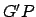is always parallel to the vector connecting the earth to the sun. It follows that a so-called conjunction, at which the sun lies directly between the planet and the earth, occurs whenever the epicyclic anomaly,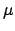, takes the value. At a conjunction, the planet is furthest from the earth, and has the same ecliptic longitude as the sun, and is, therefore, invisible. Conversely, a so-called opposition, at which the earth lies directly between the planet and the sun, occurs whenever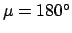. At an opposition, the planet is closest to the earth, and also directly opposite the sun in the sky, and, therefore, at its brightest. Now, a superior planet rotates around the epicycle at a faster angular velocity than its guide-point rotates around the deferent. Moreover, both the planet and guide-point rotate in the same direction. It follows that the planet is traveling backward in the sky (relative to the direction of its mean motion) at opposition. This phenomenon is called retrograde motion. The period of retrograde motion begins and ends at stations--so-called because when the planet reaches them it appears to stand still in the sky for a few days whilst it reverses direction.

Tables 46-48 can be used to determine the dates of the conjunctions, oppositions, and stations of Mars. Consider the first conjunction after the epoch (January 1, 2000 CE). We can estimate the time at which this event occurs by approximating the epicyclic anomaly as the so-called mean epicyclic anomaly: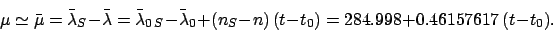We obtain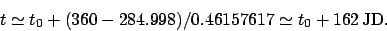A calculation of the epicyclic anomaly at this time, using Tables 46-48, yields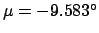. Now, the actual conjunction occurs when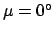. Hence, our next estimate is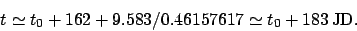A calculation of the epicyclic anomaly at this time gives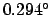. Thus, our final estimate is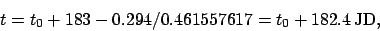which corresponds to July 1, 2000 CE.

Consider the first opposition of Mars after the epoch. Our first estimate of the time at which this event takes place is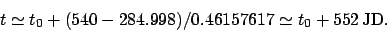A calculation of the epicyclic anomaly at this time yields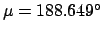. Now, the actual opposition occurs when. Hence, our second estimate is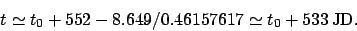A calculation of the epicyclic anomaly at this time gives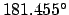. Thus, our third estimate is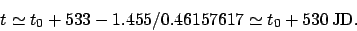A calculation of the epicyclic anomaly at this time yields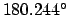. Hence, our final estimate is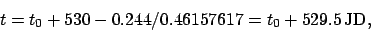which corresponds to June 13, 2001 CE. Incidentally, it is clear from the above analysis that the mean time period between successive conjunctions, or oppositions, of Mars is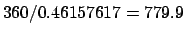JD, which is equivalent to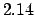years.

Let us now consider the stations of Mars. We can approximate the ecliptic longitude of a superior planet as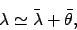(184)

where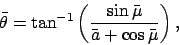(185)

and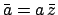. Note that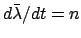and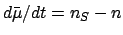. It follows that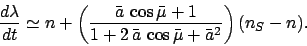(186)

Now, a station corresponds to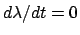(i.e., a local maximum or minimum of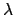), which gives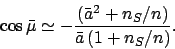(187)

For the case of Mars, we find that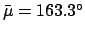or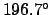. The first solution corresponds to the so-called retrograde station, at which the planet switches from direct to retrograde motion. The second solution corresponds to the so-called direct station, at which the planet switches from retrograde to direct motion. The mean time interval between a retrograde station and the following opposition, or between an opposition and the following direct station, is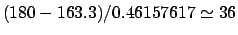JD. Unfortunately, the only option for accurately determining the dates at which the stations occur is to calculate the ecliptic longitude of Mars over a range of days centered 36 days before and after its opposition.

Table 49 shows the conjunctions, oppositions, and stations of Mars for the years 2000-2020 CE, calculated using the techniques described above.Next: Jupiter Up: The Superior Planets Previous: Mars
Richard Fitzpatrick 2010-07-21